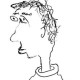# Remarks on Fargues-Scholze, part 2

Today I want to talk about section VII.3 in the manuscript. Here they define and study a functor$f_{\natural}$ on solid sheaves which is left adjoint to the usual pullback functor. But why is this called relative homology?

To explain this name, you have to remember that in the usual formalism of etale cohomology, homology is realized as the compactly supported cohomology of the dualizing complex. Symbolically, if$X$ is a variety with structure map$f:X \to \mathrm{Spec} k$, then the homology of$X$ is given by$Rf_! Rf^! \Lambda$. Now, you might ask whether this works in families: if$f:X \to Y$ is some map of varieties, maybe I can find some complex on$Y$ whose stalk at$y$ realizes the homology of$X_y$? If the constant sheaf is$f$-ULA, then formation of$Rf^! \Lambda$ commutes with any base change, and exactly the same formula works, but in general there is no naive sheaf with this property.

The punchline now is that$f_{\natural} \Lambda$ does have this property: when the constant sheaf is$f$-ULA (e.g. if$Y$ is a point) it agrees with$Rf_! Rf^! \Lambda$ by Proposition VII.5.2, and its formation commutes with arbitrary base change, so it really does give a complex on$Y$ whose stalks realize the homology of the fibers of$f$. The only twist is that$f_{\natural}\Lambda$ is a solid sheaf in general, not a classical etale sheaf.

Posted on Categories Math

## 3 thoughts on “Remarks on Fargues-Scholze, part 2”

1.Peter Scholze says:

I think you have this backwards!

More precisely, I claim that being a left adjoint to pullback$f^\ast$ means *a priori* that this is relative homology, just like the right adjoint to pullback is relative cohomology. For example, if$X$ is any anima, and$\pi: X\to \ast$ is the projection to a point, then one has a pullback functor$\pi^\ast: D(\mathbb{Z})\to D(X,\mathbb{Z})$, and this admits a left adjoint$\pi_{\natural}$, with its value on the constant sheaf$\mathbb{Z}$ being$\pi_{\natural} \mathbb{Z}=H_\bullet(X,\mathbb{Z})$, the homology of$X$.

The identification of$f_{\natural} f^{\ast}$ with$Rf_! Rf^!$ in some examples of interest is then an a posteriori justification for calling the other thing etale homology.

Like

1.arithmetica says:

Thanks for offering this alternative perspective! It’s an easy exercise that over a point,$Rf_! Rf^! \Lambda$ is the$\Lambda$-dual of$R\Gamma(X,\Lambda)$, which I would argue is an a priori justification for calling the first thing etale homology.

Maybe I just like varieties more than I like anima…

Like

1.Dustin says:

Unsurprisingly, I think you’re both right, and actually you’re secretly saying the same thing! I’d like to elaborate a bit on why, even though what I’m saying is probably clear to both of you.

First of all, though, I don’t think this has much to do with varieties vs anima. At least not if we ignore sociology: it’s true that anima-people like their left adjoints to pullback, while varieties-people never consider them, or at least not explicitly, preferring to take the relative compactly supported cohomology$f_!$ as fundamental instead of the relative homology. (And with good justification, because the former is always defined but the latter not.) But mathematically speaking, the point Peter’s making can be made with varieties, locally compact Hausdorff spaces, anima, or whatever else you like. Namely, if the left adjoint to pullback (exists and) commutes with base-change, then it’s reasonable to interpret it as in-families homology, just as if the formation of the right adjoint to pullback commutes with base-change, it’s reasonable to interpret it as in-families cohomology. In varieties-world, the first case gets called “ULA” and the second case gets called “proper”, but formally they are completely dual to one another.

That said, I do like the explanation in terms of the six functor formalism. In fact, in some sense, it also applies in the case of anima, and also in the case of solid sheaves as in Fargues-Scholze. As far as I’m concerned, to give a covariant functoriality the notation$f_!$, it is enough that it preserves colimits and commutes with pullbacks. It doesn’t have to match up with what you’d usually interpret as “compactly supported cohomology” in some given class of examples. So for proper maps of varieties you’re allowed to take$f_!=f_\ast$ and this forces the usual six-functor formalism, but in situations where the left adjoint to pullback exists and commutes with base-change (such as anima, or the solid sheaves in Fargues-Scholze), you’re allowed to take$f_!$ to be this left adjoint. (Of course there can be a consistency question if you try to do both at once, which I guess is what led Fargues-Scholze to use the alternate notation$f_\natural$.) In this situation “all maps are etale” ($f^!=f^\ast$) and your and Peter’s explanations are actually the same, because$f_!f^!=f_\natural f^\ast$.

I’ll also add that if we’re talking about ordinary sheaves (of abelian groups, say), then from the perspective of the larger world of condensed anima, it makes sense that all maps of anima should be etale, becuase anima are “discrete” in sense of having trivial condensed structure. But this is very much related to the fact that the coefficients in our sheaf theory are also discrete. When we move to the more general solid sheaves on condensed sets, for example, then all condensed sets there suddenly also count as “discrete”, because they are discrete as seen from the perspective of the coefficient systems in play.

This was a long-winded way of saying that formally speaking I think it’s reasonable to view the Fargues-Scholze formalism not as being somehow opposed or alternate to the six functor formalism, but really just a special case, where everything is viewed as etale.

Like